# Test Prep Plan - Take a practice test

Take this practice test to check your existing knowledge of the course material. We'll review your answers and create a Test Prep Plan for you based on your results.
How Test Prep Plans work
1
2Based on your results, we'll create a customized Test Prep Plan just for you!
3Study smarter
Study more effectively: skip concepts you already know and focus on what you still need to learn.

# NMTA Math: Applications of Integration Chapter Exam

Exam Instructions:

Choose your answers to the questions and click 'Next' to see the next set of questions. You can skip questions if you would like and come back to them later with the yellow "Go To First Skipped Question" button. When you have completed the practice exam, a green submit button will appear. Click it to see your results. Good luck!

### Page 1

#### Question 2 2. Given the cut-off cone pictured, with h = x+3 and the region extending from x=2 to x=4, what is the integral you will need to solve to find the volume of this cut-off cone?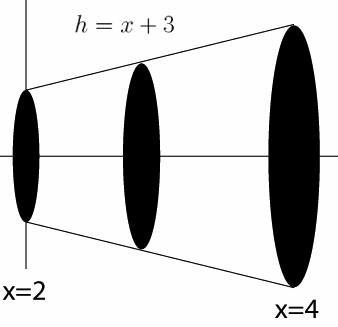#### Question 3 3. Given a velocity v(t) as defined below and a starting position of x=1 at t=0, what is the equation for your position as a function of time?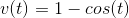#### Question 4 4. What is the equation for the volume of this cone that extends from x=0 to x=A, given that h=x?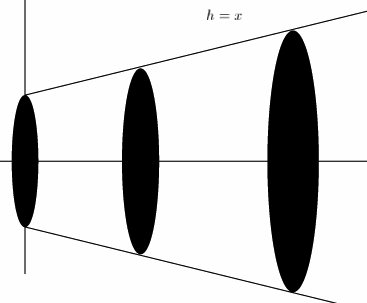#### Question 5 5. What is the equation for the volume of this cone that extends from x=0 to x=A, given that h=x?### Page 2

#### Question 7 7. If your velocity is given by v(t), and you start at x=4 at t=0, then for what value of t will you be at the position x=20?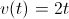#### Question 8 8. What is the volume made by revolving f(x) around the x-axis between x=0 and x=1?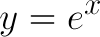#### Question 10 10. Given a velocity of v(t) as defined below, what is the equation for your position as a function of time?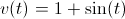### Page 3

#### Question 12 12. What is the volume made by revolving the area between g(x) and f(x) around the x-axis between x=0 and x=1?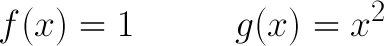#### Question 13 13. What is the equation for the volume of this cone that extends from x=0 to x=A, given that h=x?#### Question 14 14. What is the volume made by revolving f(x) around the x-axis between x=0 and x=1?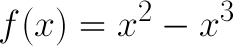#### Question 15 15. What is the volume of the cut-off cone shown?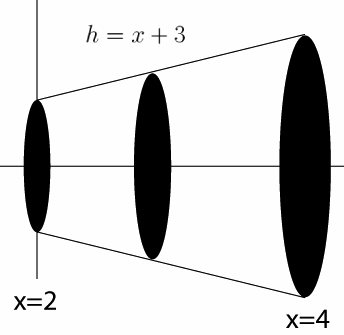#### NMTA Math: Applications of Integration Chapter Exam Instructions

Choose your answers to the questions and click 'Next' to see the next set of questions. You can skip questions if you would like and come back to them later with the yellow "Go To First Skipped Question" button. When you have completed the practice exam, a green submit button will appear. Click it to see your results. Good luck!

Support